## How to Calculate and Solve for Linear Shrinkage | Solidification of Metals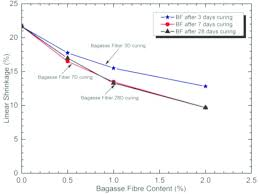The image above represents linear shrinkage.

To compute for linear shrinkage, two essential parameters are needed and these parameters are Linear Dimension of the Mould (lm) and Size of the Casting at 20°C (lo).

The formula for calculating linear shrinkage:

εL = lm – lo / lo x 100%

Where:

εL = Linear Shrinkage
lm = Linear Dimension of the Mould
lo = Size of Casting at 20°C

Let’s solve an example;
Find the Linear shrinkage where the linear dimension of the mould is 20 and the size of casting is 10.

This implies that;

lm = Linear Dimension of the Mould = 20
lo = Size of Casting at 20°C = 10

εL = lm – lo / lo x 100%
εL = 20 – 10 / 10 x 100%
εL = 10 / 10 x 100%
εL = 1 x 100%
εL = 100%

Therefore, the linear shrinkage is 100%.

## How to Calculate and Solve for Heat Flux | Solidification of Metals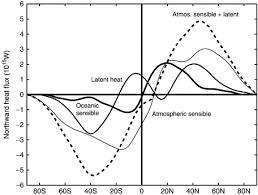The image above represents heat flux.

To compute for heat flux, three essential parameters are needed and these parameters are Heat Emission (h), Surface Temperature (Ts) and Initial Temperature (To).

The formula for calculating heat flux:

qx = h(Ts – To)

Where:

qx = Heat Flux (T)
h = Heat Emission
Ts = Surface Temperature
To = Initial Temperature

Let’s solve an example;
Find the heat flux when the heat emission is 21, the surface temperature is 12 and the initial temperature is 10.

This implies that;

h = Heat Emission = 21
Ts = Surface Temperature = 12
To = Initial Temperature = 10

qx = h(Ts – To)
qx = 21(12 – 10)
qx = 21(2)
qx = 42

Therefore, the heat flux is 42 W.

Calculating the Heat Emission when the Heat Flux, the Surface Temperature and the Initial Temperature is Given.

h = qx / Ts – To

Where:

h = Heat Emission
qx = Heat Flux (T)
Ts = Surface Temperature
To = Initial Temperature

Let’s solve an example;
Find the heat emission when the heat flux is 40, the surface temperature is 20 and the initial temperature is 10.

This implies that;

qx = Heat Flux (T) = 40
Ts = Surface Temperature = 20
To = Initial Temperature = 10

h = qx / Ts – To
h = 40 / 20 – 10
h = 40 / 10
h = 4

Therefore, the heat emission is 4.

## How to Calculate and Solve for Freezing Time | Solidification of Metals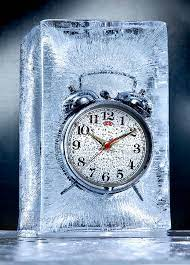The image above represents freezing time.

To compute for freezing time, three essential parameters are needed and these parameters are Thickness of Solidified Metal (M), Constant (β) and Heat Diffusivity (α).

The formula for calculating freezing time:

t = / 4β²α

Where:

t = Freezing Time
M = Thickness of Solidified Metal
β = Constant
α = Heat Diffusivity

Let’s solve an example;
Find the freezing time when the thickness of solidified metal is 8, the constant is 10 and the heat diffusivity is 2.

This implies that;

M = Thickness of Solidified Metal = 8
β = Constant = 10
α = Heat Diffusivity = 2

t = / 4β²α
t = / 4(10)²(2)
t = 64 / 4(100)(2)
t = 64 / 800
t = 0.08

Therefore, the freezing time is 0.08 s.

Calculating the Thickness of Solidified Metal when the Freezing Time, the Constant and the Heat Diffusivity is Given.

M = t x 4β²α

Where:

M = Thickness of Solidified Metal
t = Freezing Time
β = Constant
α = Heat Diffusivity

Let’s solve an example;
Find the thickness of solidified metal when the freezing time is 21, the constant is 9 and the heat diffusivity is 2.

This implies that;

t = Freezing Time = 21
β = Constant = 9
α = Heat Diffusivity = 2

M = t x 4β²α
M = 21 x 4(9²)(2)
M = 21 x 4(81)(2)
M = 21 x 4(162)
M = 21 x 648
M = 13668

Therefore, the thickness of solidified metal is 13668.

## How to Calculate and Solve for Effective Heat of Fusion | Solidification of Metals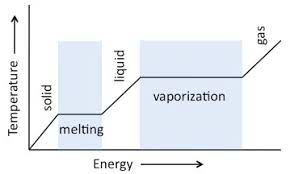The image above represents effective heat of fusion.

To compute for effective heat of fusion, three essential parameters are needed and these parameters are Latent Heat of Fusion (Hf), Heat Capacity at Constant Pressure (cp) and Change in Temperature (ΔT).

The formula for calculating effective heat of fusion:

H = Hf + cpΔT

Where:

H = Effective Heat of Fusion
Hf = Latent Heat of Fusion
cp = Heat Capacity at Constant Pressure
ΔT = Change in Temperature

Let’s solve an example;
Find the effective heat of fusion when the latent heat of fusion is 12, the heat capacity at constant pressure is 14 and the change in temperature is 10.

This implies that;

Hf = Latent Heat of Fusion = 12
cp = Heat Capacity at Constant Pressure = 14
ΔT = Change in Temperature = 10

H = Hf + cpΔT
H = 12 + 14(10)
H = 12 + 140
H = 152

Therefore, the effective heat of fusion is 152 J/Kg.

Calculating the Latent Heat of Fusion when the Effective Heat of Fusion, the Heat Capacity at Constant Pressure and the Change in Temperature is Given.

Hf = H – cpΔT

Where:

Hf = Latent Heat of Fusion
H = Effective Heat of Fusion
cp = Heat Capacity at Constant Pressure
ΔT = Change in Temperature

Let’s solve an example;
Find the latent heat of fusion when the effective heat of fusion is 42, the heat capacity at constant pressure is 10 and the change in temperature is 2.

This implies that;

H = Effective Heat of Fusion = 42
cp = Heat Capacity at Constant Pressure = 10
ΔT = Change in Temperature = 2

Hf = H – cpΔT
Hf = 42 – (10)(2)
Hf = 42 – 20
Hf = 22

Therefore, the latent heat of fusion is 22.

## How to Calculate and Solve for Total Latent Heat | Solidification of Metals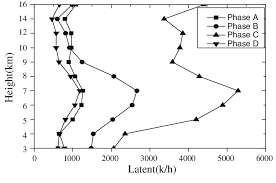The image above represents total latent heat.

To compute for total latent heat, three essential parameters are needed and these parameters are Density of Solidifying Metal (ρ’), Volume of Casting (V) and Latent Heat of Fusion (Hf).

The formula for calculating the total latent heat:

Q = ρ’VHf

Where:

Q = Total Latent Heat
ρ = Density of Solidifying Metal
V = Volume of Casting
Hf = Latent Heat of Fusion

Let’s solve an example;
Find the total latent heat when the density of solidifying metal is 12, the volume of casting is 14 and the latent heat of fusion is 10.

This implies that;

ρ = Density of Solidifying Metal = 12
V = Volume of Casting = 14
Hf = Latent Heat of Fusion = 10

Q = ρ’VHf
Q = (12)(14)(10)
Q = 1680

Therefore, the total latent heat is 1680 J/Kg.

Calculating the Density of Solidifying Metal when the Total Latent Heat, the Volume of Casting and the Latent Heat of Fusion is Given.

ρ = Q / VHf

Where;

ρ = Density of Solidifying Metal
Q = Total Latent Heat
V = Volume of Casting
Hf = Latent Heat of Fusion

Let’s solve an example;
Find the density of solidifying metal when the total latent heat is 20, the volume of casting is 4 and the latent heat of fusion is 2.

This implies that;

Q = Total Latent Heat = 20
V = Volume of Casting = 4
Hf = Latent Heat of Fusion = 2

ρ = Q / VHf
ρ = 20 / (4)(2)
ρ = 20 / 8
ρ = 2.5

Therefore, the density of solidifying metal is 2.5

## How to Calculate and Solve for Rate at Which Latent Heat is Evolved | Solidification of Metals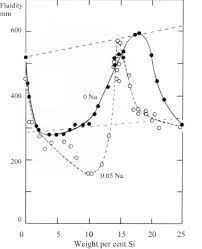The image above represents rate at which latent heat is evolved.

To compute for rate at which latent heat is evolved, three essential parameters are needed and these parameters are Density of Solidifying Metal (ρ’), Latent Heat of Fusion of Metal (Hf) and Mass Flow Rate (dM/dt).

The formula for calculating rate at which latent heat is evolved:

qx = ρ’Hf(dM/dt)

Where:

qx = Rate at Which Latent Heat is Evolved
ρ’ = Density of Solidifying Metal
Hf = Latent Heat of Fusion of Metal
dM/dt = Mass Flow Rate

Let’s solve an example;
Find the rate at which latent heat is evolved when the density of solidifying metal is 8, the latent heat of fusion of metal is 4 and the mass flow rate is 2.

This implies that;

ρ’ = Density of Solidifying Metal = 8
Hf = Latent Heat of Fusion of Metal = 4
dM/dt = Mass Flow Rate = 2

qx = ρ’Hf(dM/dt)
qx = (8)(4)(2)
qx = 64

Therefore, the rate at which latent heat is evolved is 64.

Calculating the Density of Solidifying Metal when the Rate at which Latent Heat is Evolved, the Latent Heat of Fusion of Metal and the Mass Flow Rate is Given.

ρ’ = qx / Hf (dM/dt)

Where;

ρ’ = Density of Solidifying Metal
qx = Rate at Which Latent Heat is Evolved
Hf = Latent Heat of Fusion of Metal
dM/dt = Mass Flow Rate

Let’s solve an example;
Find the density of solidifying metal when the rate at which latent heat is evolved is 25, the latent heat of fusion of metal is 5 and the mass flow rate is 3.

This implies that;

qx = Rate at Which Latent Heat is Evolved = 25
Hf = Latent Heat of Fusion of Metal = 5
dM/dt = Mass Flow Rate = 3

ρ’ = qx / Hf (dM/dt)
ρ’ = 25 / 5 (3)
ρ’ = 25 / 15
ρ’ = 1.67

Therefore, the density of solidifying metal is 1.67.
Continue reading How to Calculate and Solve for Rate at Which Latent Heat is Evolved | Solidification of Metals

## How to Calculate and Solve for Heat Diffusivity | Solidification of Metals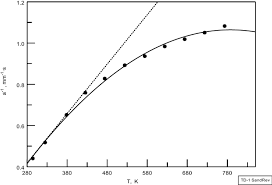The image above represents heat diffusivity.

To compute for heat diffusivity, three essential parameters are needed and these parameters are Thermal Conductivity (K), Density (ρ) and Heat Capacity at Constant Pressure (cp).

α = Kρcp

Where:

α = Heat Diffusivity
K = Thermal Conductivity
ρ = Density
cp = Heat Capacity at Constant Pressure

Let’s solve an example;
Find the heat diffusivity when the thermal conductivity is 10, the density is 12 and the heat capacity at constant pressure is 18.

This implies that;

K = Thermal Conductivity = 10
ρ = Density = 12
cp = Heat Capacity at Constant Pressure = 18

α = Kρcp
α = (10)(12)(18)
α = 2160

Therefore, the heat diffusivity is 2160 m²/s.

Calculating the Thermal Conductivity when the Heat Diffusivity, the Density and the Heat Capacity at Constant Pressure is Given.

K = α / ρcp

Where:

K = Thermal Conductivity
α = Heat Diffusivity
ρ = Density
cp = Heat Capacity at Constant Pressure

Let’s solve an example;
Find the thermal conductivity when the heat diffusivity is 15, the density is 10 and the heat capacity at constant pressure is 14.

This implies that;

α = Heat diffusivity = 15
ρ = Density = 10
cp = Heat Capacity at Constant Pressure = 14

K = α / ρcp
K = 15 / 10 (14)
K = 15 / 140
K = 0.107

Therefore, the thermal conductivity is 0.107.

Calculating the Density when the Heat Diffusivity, the Thermal Conductivity and the Heat Capacity at Constant Pressure is Given.

ρ = α / K x cp

Where:

ρ = Density
α = Heat Diffusivity
K = Thermal Conductivity
cp = Heat Capacity at Constant Pressure

Let’s solve an example;
Find the density when the heat diffusivity is 18, the thermal conductivity is 12 and the heat capacity at constant pressure is 4.

This implies that;

α = Heat Diffusivity = 18
K = Thermal Conductivity = 12
cp = Heat Capacity at Constant Pressure = 4

ρ = α / K x cp
ρ = 18 / 12 x 4
ρ = 18 / 48
ρ = 0.375

Therefore, the density is 0.375.

Calculating the Heat Capacity at Constant Pressure when the Heat Diffusivity, the Thermal Conductivity and the Density is Given.

cp = α / K x ρ

Where:

cp = Heat Capacity at Constant Pressure
α = Heat Diffusivity
K = Thermal Conductivity
ρ = Density

Let’s solve an example;
Find the heat capacity at constant pressure when the heat diffusivity is 10, the thermal conductivity is 20 and the density is 6.

This implies that;

α = Heat Diffusivity = 10
K = Thermal Conductivity = 20
ρ = Density = 6

cp = α / K x ρ
cp = 10 / 20 x 6
cp = 10 / 120
cp = 0.083

Therefore, the heat capacity at constant pressure is 0.083.

Nickzom Calculator – The Calculator Encyclopedia is capable of calculating the heat diffusivity.

To get the answer and workings of the heat diffusivity using the Nickzom Calculator – The Calculator Encyclopedia. First, you need to obtain the app.

You can get this app via any of these means:

To get access to the professional version via web, you need to register and subscribe for NGN 2,000 per annum to have utter access to all functionalities.
You can also try the demo version via https://www.nickzom.org/calculator

Apple (Paid) – https://itunes.apple.com/us/app/nickzom-calculator/id1331162702?mt=8
Once, you have obtained the calculator encyclopedia app, proceed to the Calculator Map, then click on Materials and Metallurgical under Engineering.Now, Click on Solidification of Metal under Materials and Metallurgical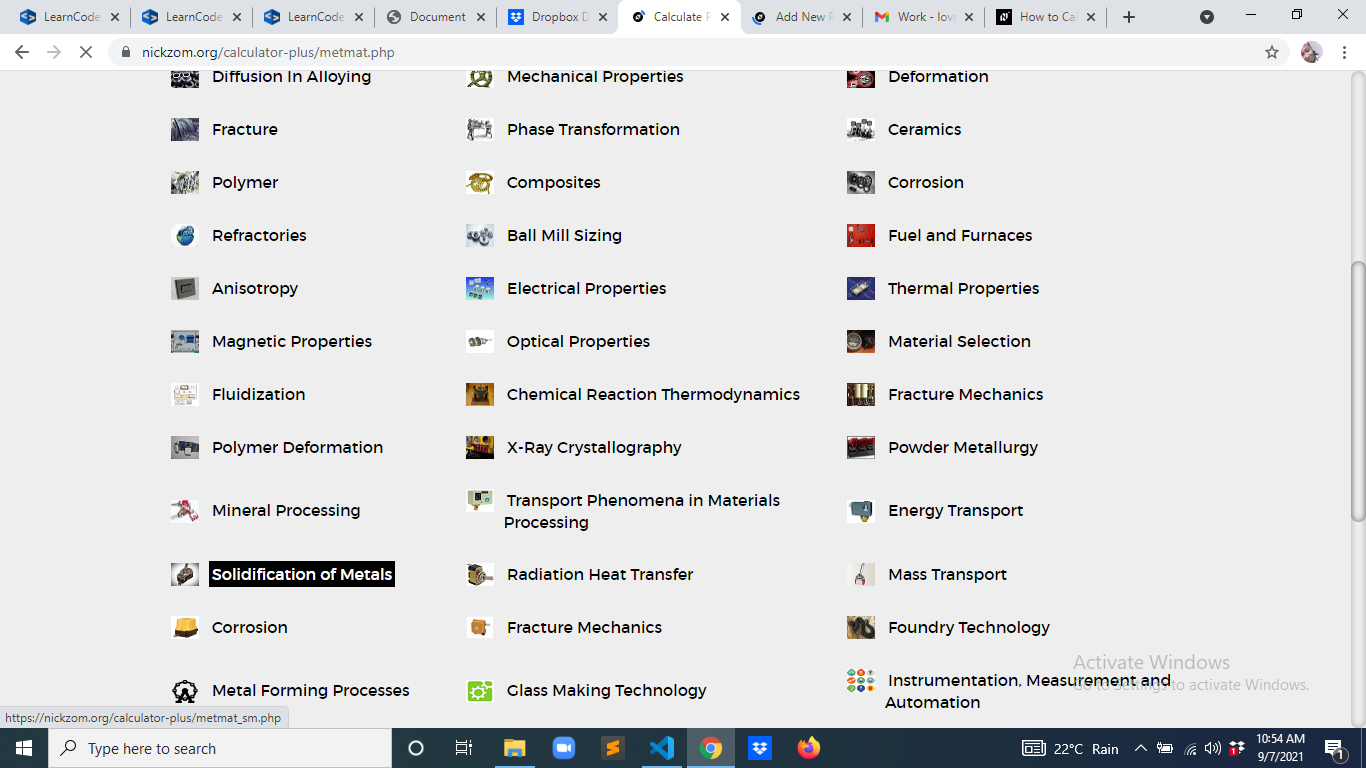Now, Click on Heat Diffusivity under Solidification of Metal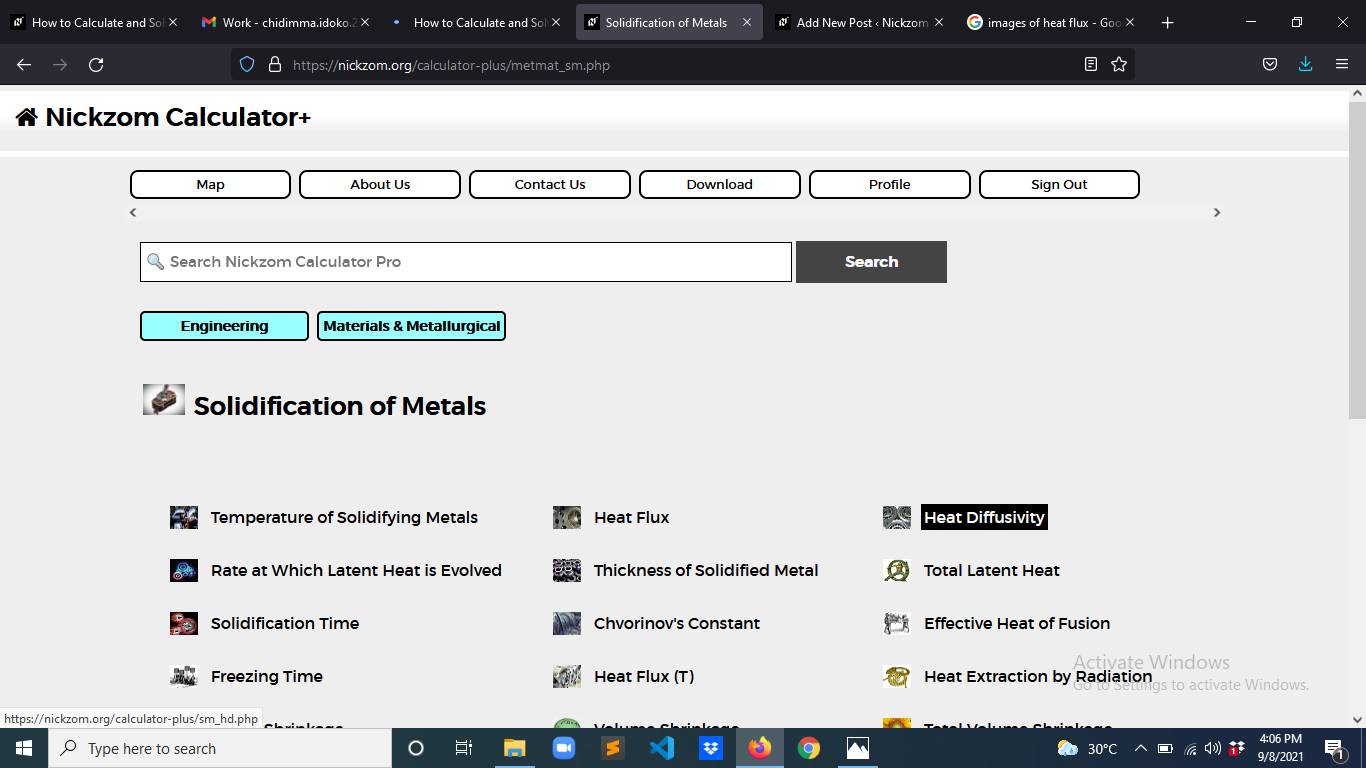The screenshot below displays the page or activity to enter your values, to get the answer for the heat diffusivity according to the respective parameter which is the Thermal Conductivity (K), Density (ρ) and Heat Capacity at Constant Pressure (cp).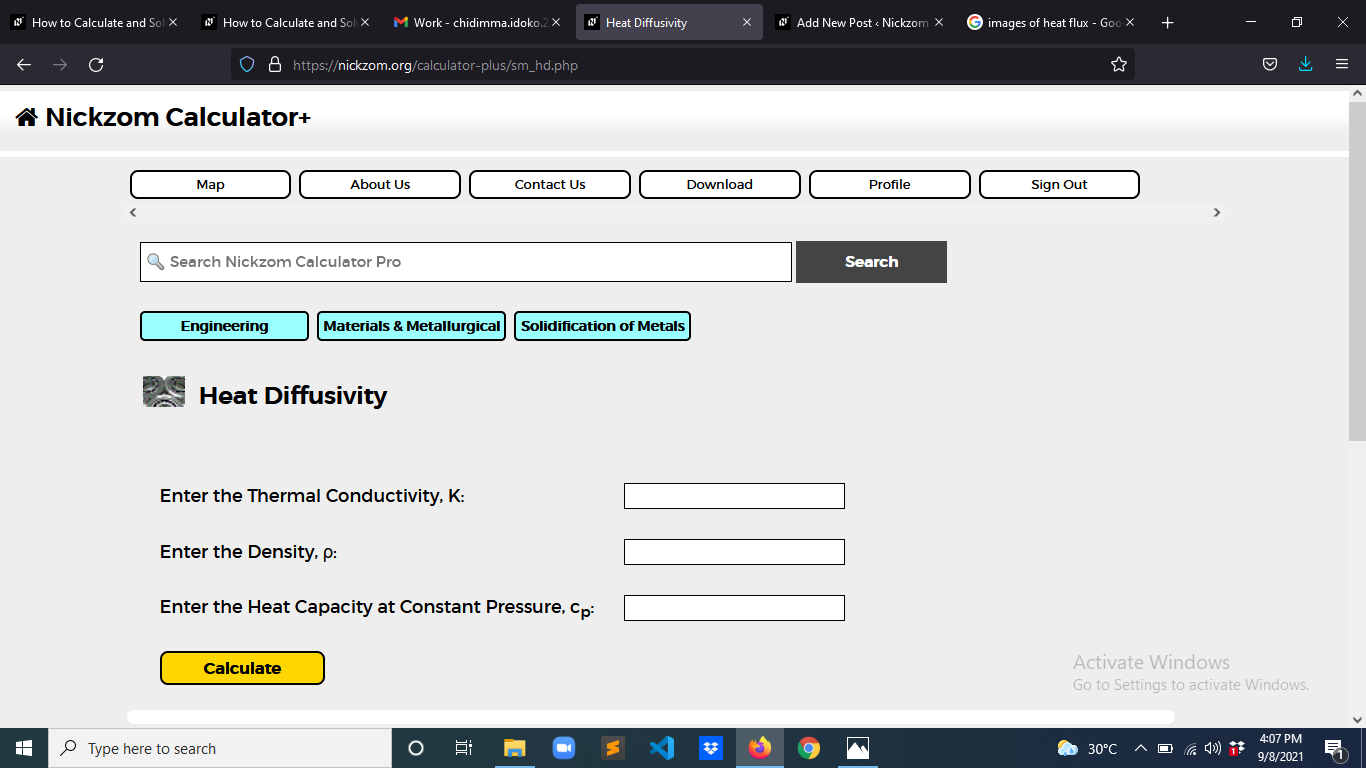Now, enter the values appropriately and accordingly for the parameters as required by the Thermal Conductivity (K) is 10, Density (ρ) is 12 and Heat Capacity at Constant Pressure (cp) is 18.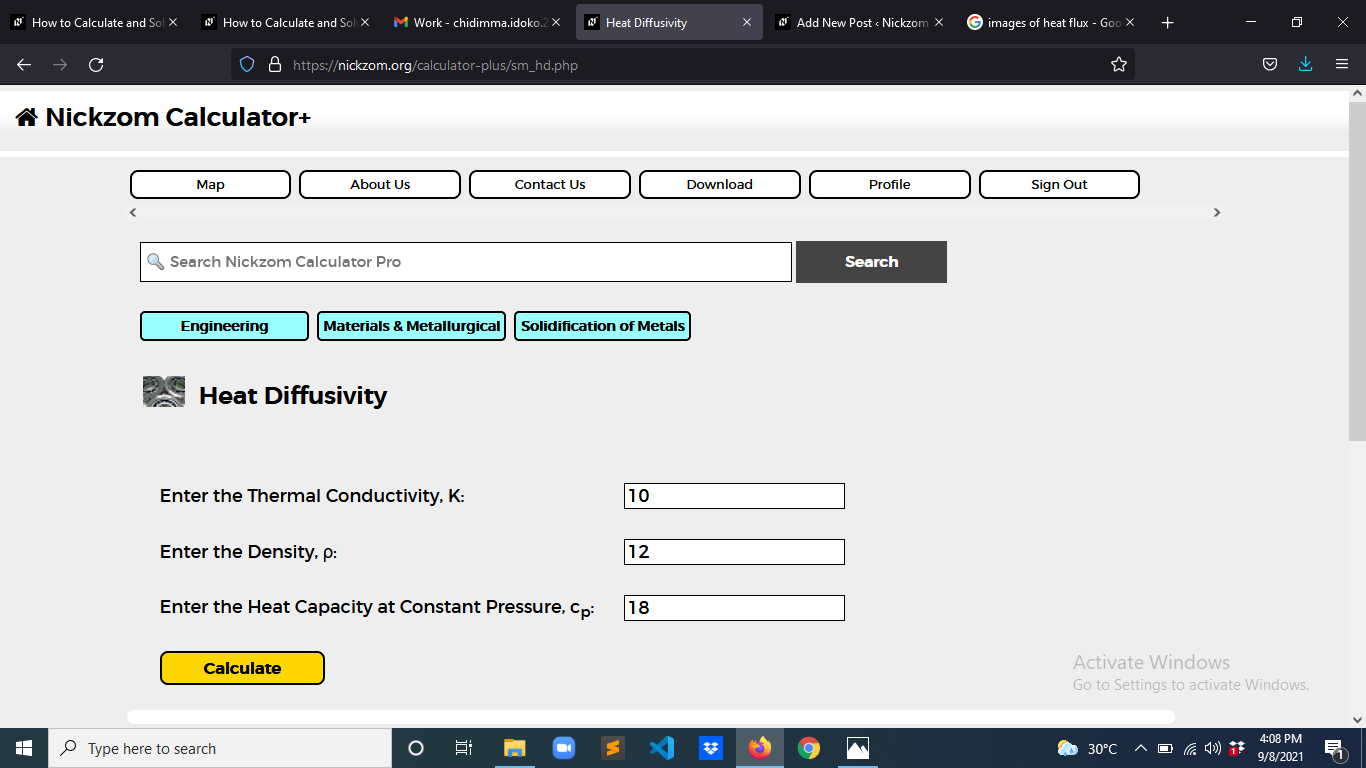Finally, Click on Calculate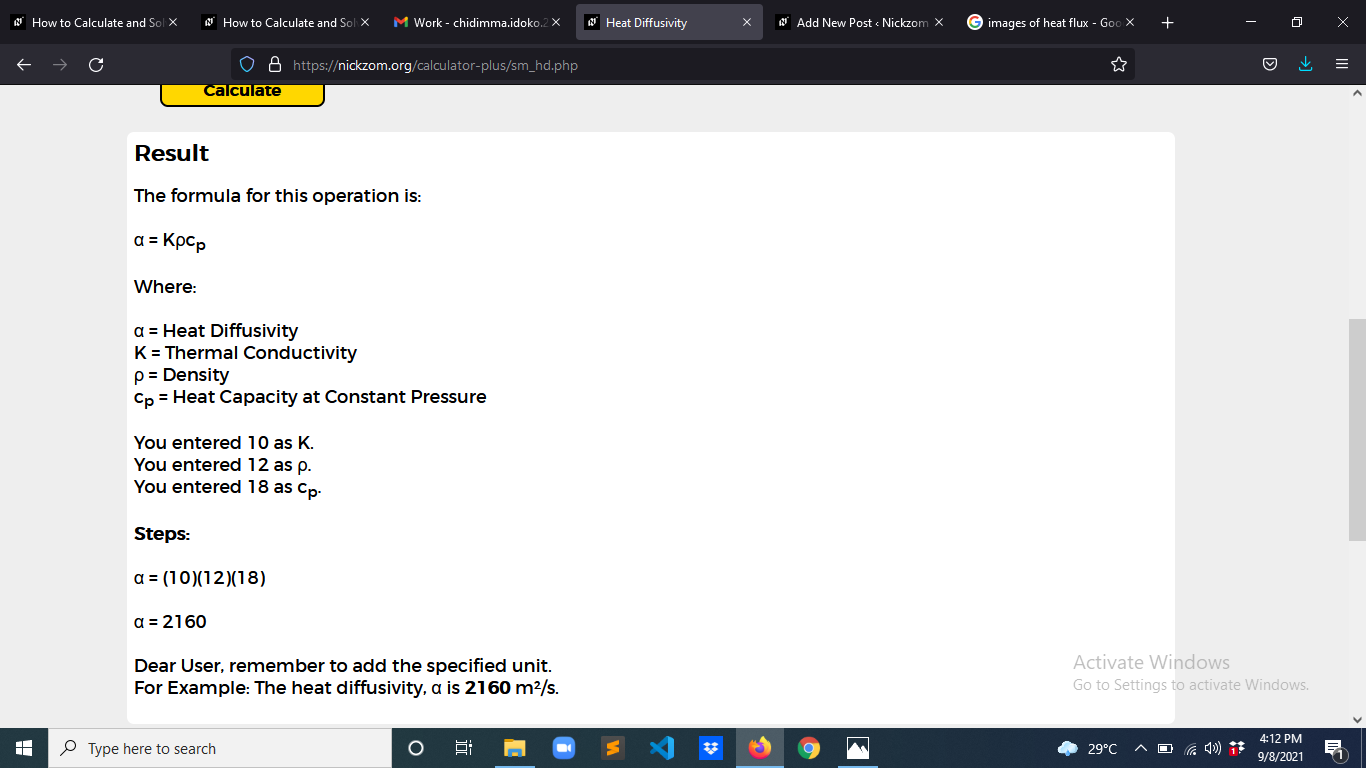As you can see from the screenshot above, Nickzom Calculator– The Calculator Encyclopedia solves for the heat diffusivity and presents the formula, workings and steps too.

## How to Calculate and Solve for Heat Flux | Solidification of Metals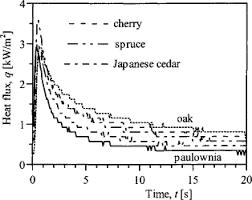The image above represents heat flux.

To compute for heat flux, three essential parameters are needed and these parameters are Thermal Conductivity (K), Density (ρ) and Heat Capacity at Constant Pressure (cp).

The formula for calculating heat flux:

q = K / ρcp

Where:

q = Heat Flux
K = Thermal Conductivity
ρ = Density
cp = Heat Capacity at Constant Pressure

Let’s solve an example;
Find the heat flux when the thermal conductivity is 21, the density is 12 and the heat capacity at constant pressure is 24.

This implies that;’

K = Thermal Conductivity = 21
ρ = Density = 12
cp = Heat Capacity at Constant Pressure = 24

q = K / ρcp
q = 21 / (12)(24)
q = 21 / 288
q = 0.0729

Therefore, the heat flux is 0.0729 W.

Calculating the Thermal Conductivity when the Heat Flux, the Density and the Heat Capacity at Constant Pressure is Given.

K = q (ρ cp)

Where:

K = Thermal Conductivity
q = Heat Flux
ρ = Density
cp = Heat Capacity at Constant Pressure

Let’s solve an example;
Find the thermal conductivity when the heat flux is 15, the density is 10 and the heat capacity at constant pressure is 14.

This implies that;

q = Heat Flux = 15
ρ = Density = 10
cp = Heat Capacity at Constant Pressure = 14

K = q (ρ cp)
K = 15 (10 x 14)
K = 15 (140)
K = 2100

Therefore, the thermal conductivity is 2100.